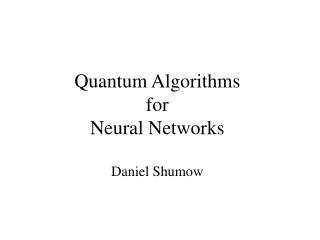DownloadDownload PresentationQuantum Algorithms for Neural Networks Daniel Shumow

Quantum Algorithms for Neural Networks Daniel Shumow

Download PresentationQuantum Algorithms for Neural Networks Daniel Shumow

- - - - - - - - - - - - - - - - - - - - - - - - - - - E N D - - - - - - - - - - - - - - - - - - - - - - - - - - -
Presentation Transcript

1. Quantum AlgorithmsforNeural NetworksDaniel Shumow

2. Outline • Project Motivation • Brief overview of Quantum Concepts • Linear superposition • Interference and Entanglement • Quantum Computation • Quantum Neural Algorithms • Quantum Associative Memory

3. Motivation for Project • By using components that take advantage of the laws of Quantum Mechanics it has been shown that there are theoretical algorithmic performance improvements not possible from classical computers. • Can Quantum Computation be used to improve the performance of Neural Network Algorithms?

4. Quantum Mechanics • Quantum Systems can be in more than one state at once. This is called a super position of states. • Quantum systems are described by a wave function often denoted by the Greek letter  (psi) • For state x: (x) evaluates to a complex number such that (x)·(x)* is the probability that the quantum system will collapse into state x when it interacts with the environment. • Wave functions evolve by unitary transformations.

5.  can be represented as a column vector.  is a normalized linear combination of basis states. When  interacts with the environment it is projected onto a basis state. Quantum Mechanics:Linear Superposition Where cj is complex and:

6. Quantum MechanicsInterference and Entanglement • Interference:States that are in a super position can interfere with each other causing probability amplitudes to increase or decrease. This is like water waves interfering. • Entanglement:A purely quantum phenomenon, entanglement is when changes to one part of a quantum system instantaneously correlate at another part of the quantum system. Young’s double slit experiment:

7. Quantum Computation • Quantum algorithms get power from superposition, interference, and entanglement. • In a quantum computer the registers will be quantum systems. • The two “big” quantum algorithms are: • Shor’s Algorithm for Factoring: Factors composite integers in polynomial time. • Grover’s Searching Algorithm: Provides a square root speed up over classical algorithms for searching unordered lists.

8. Quantum-Neural Algorithms • Quantum Associative Memory • Ventura and Martinez, 1998. • Competitive Learning in a Quantum System • Ventura, 1999.

9. Quantum Associative Memory(QuAM) A QuAM is analogous to a linear associative memory. It is a neural network that has an input layer and an output layer. All neurons are quantum mechanical components.

10. QuAMProperties • The whole network is a quantum system. • Neurons do not have to be individually updated because the system will update itself. • A QuAM can store an exponential number of patterns with perfect recall. • A QuAM can generalize but it is worse than classical algorithms when generalizing.

11. QuAMTraining Algorithm • Training samples consist of an input pattern and a desired output pattern. • A wave function is designed such that the input patterns are entangled to the corresponding output patterns. • The wave function is set up so when a pattern is applied to the network the correct answer constructively interferes and incorrect answers destructively interfere with each other.

12. QuAMResults